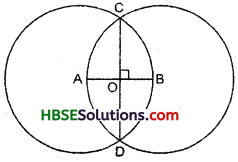# HBSE 6th Class Maths Solutions Chapter 14 Practical Geometry Ex 14.1

Haryana State Board HBSE 6th Class Maths Solutions Chapter 14 Practical Geometry Ex 14.1 Textbook Exercise Questions and Answers.

## 14.1

Question 1.
Draw a circle of radius 3.2 cm.
Solution:
Steps of construction :
(i) Open the compasses for the required radius 3.2 cm.
(ii) Mark a point ‘0’ with a sharp pencil where we want the centre of the circle to be.
(iii) Place the pointer of the compasses on 0.
(iv) Turn the compasses slowly to draw the circle.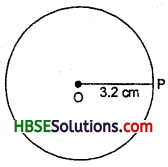Question 2.
With the same circle O, draw two circles of radii 4 cm and 2.5 cm.
Solution:
Steps of construction :
(i) Mark a point ‘O’ with a sharp pencil where we want the centre of the circle.
(ii) Open the compasses 4 cm.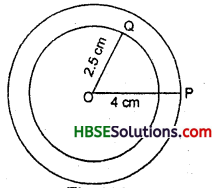(iii) Place the pointer of the compasses on O.
(iv) Turn the compasses slowly to draw the circle.
(v) Again open the compasses 2.5 cm
and place the pointer of the compasses at O. Turn the compasses slowly to draw the second circle.Question 3.
Draw a circle and any two of its diameters. If you join the ends of these diameters, what is the figure obtained ? What figure is obtained if the diameters are perpendicular to each other ? How do you check you answer ?
Solution:
(i) By joining the ends of two diameters, we get a rectangle. By measuring, we find
AB = CD = 3 cm,
BC = AD = 2 cm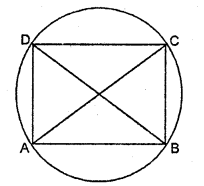i.e., pair of opposite sides are equal.
∠A = ∠B = ∠C = ∠D = 90°
i.e., each angle is equal to 90°. Hence, ABCD is a rectangle, [see Fig.]

(ii) If the diameters are perpendicular to each other, then by joining the ends of two diameters, we get a square.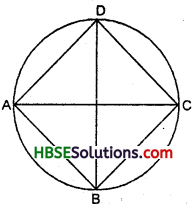By measuring, we find that
AB = BC = CD = AD = 2.5 cm
i.e., four sides are equal.
∠A = ∠B = ∠C = ∠D = 90°
i.e., each angle is a right-angle. Hence, ABCD is a square [Fig.]

Question 4.
Draw any circle and mark points A, B and C such that:
(a) A is on the circle.
(b) B is in the interior o the circle.
(c) C is in the exterior of the circle.
Solution:
(i) Mark a point‘O’with sharp pencil where we want centre of the circle.
(ii) Place the poin-ter of the compasses at ‘O’, then move the compasses slowly to draw a circle. In Fig.
(a) Point A is on the circle.
(b) Point B 1 is in the interior of the circle.
(c) Point C is in the exterior of the circle.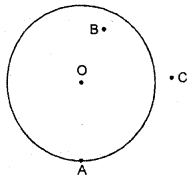Question 5.
Let A, B be the centres of two circles of equal radii, draw them so that each one of them passes through the centre of the other. Let them intersect at C and D. Examine whether $$\overline{\mathbf{A B}}$$ and $$\overline{\mathbf{CD}}$$ are at right angles.
Solution:
Draw two circles of equal radii taking A and B as their centres such that each one.
of them passes through the centre of the other. Let them intersect at C and D. Join AB and CD. [Fig.]
Yes. $$\overline{\mathbf{A B}}$$ and $$\overline{\mathbf{CD}}$$ are at right angles, because ∠BOC = 90°.